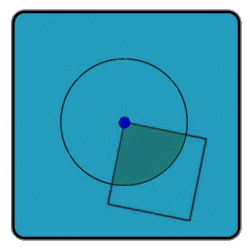All about flooble | fun stuff | Get a free chatterbox | Free JavaScript | Avatarsperplexus dot infoCircular Logic (Posted on 2002-07-03)A name which has nothing to do with this question (well almost nothing)

What percentage of the circle is shaded? (The quadrangle shown in the picture is a square with one of the vertices in the circle's center.)See The Solution Submitted by qball Rating: 1.6333 (30 votes)Comments: ( Back to comment list | You must be logged in to post comments.)re: If 25pc of the Square is shaded...| Comment 25 of 26 |(In reply to If 25pc of the Square is shaded... by imre bokor)

The shaded area, in terms of the radius of the circle, is (pi * r^2)/4.

If it's also 25% of the area of the square, then in terms of the side of the square it is (s^2)/4.

Setting these equal shows that r is equal to s / sqrt(pi).

So if s = 1 meter, then r = 1 / sqrt(pi) ~= 0.5642.

Now, if you could construct a square that had the same area as the circle, that would be something!

Edited on March 14, 2014, 9:48 pm
 Posted by tomarken on 2014-03-14 15:32:25Please log in:

 Search: Search body:
Forums (6)